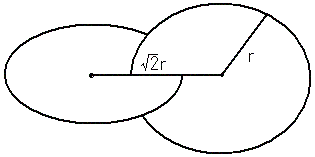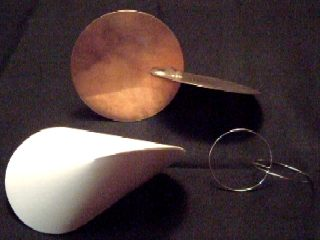Oloid Home > Geometric toy > OloidLet two disks of same size intersect and have a diameter in common. Then if the distance of two centers of disk is square root 2 times their radius ,the distance from the center of gravity to floor is constant,thus the object rolls smoothly. This object is called Two circle roller. If the distance of two centers of disk is equal to the radius then convex hull is called Oloid.(Paul Schatz1898-1979)Models of Two circle roller It is possible to make two circle roller with one wire. Animation of Two circle roller(537K) Animation of Oloid(551K) [up] [Home] [Geometric toy] [Polyhedral toy] [Java Applet] [Links] [What's New]